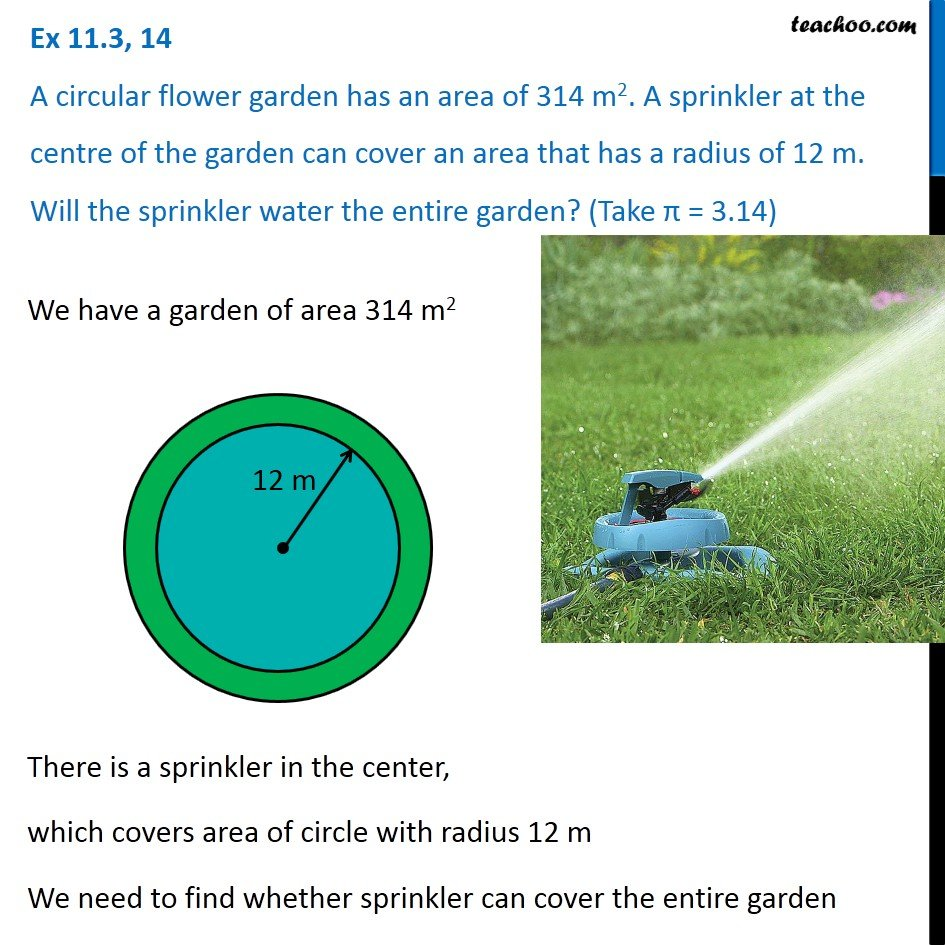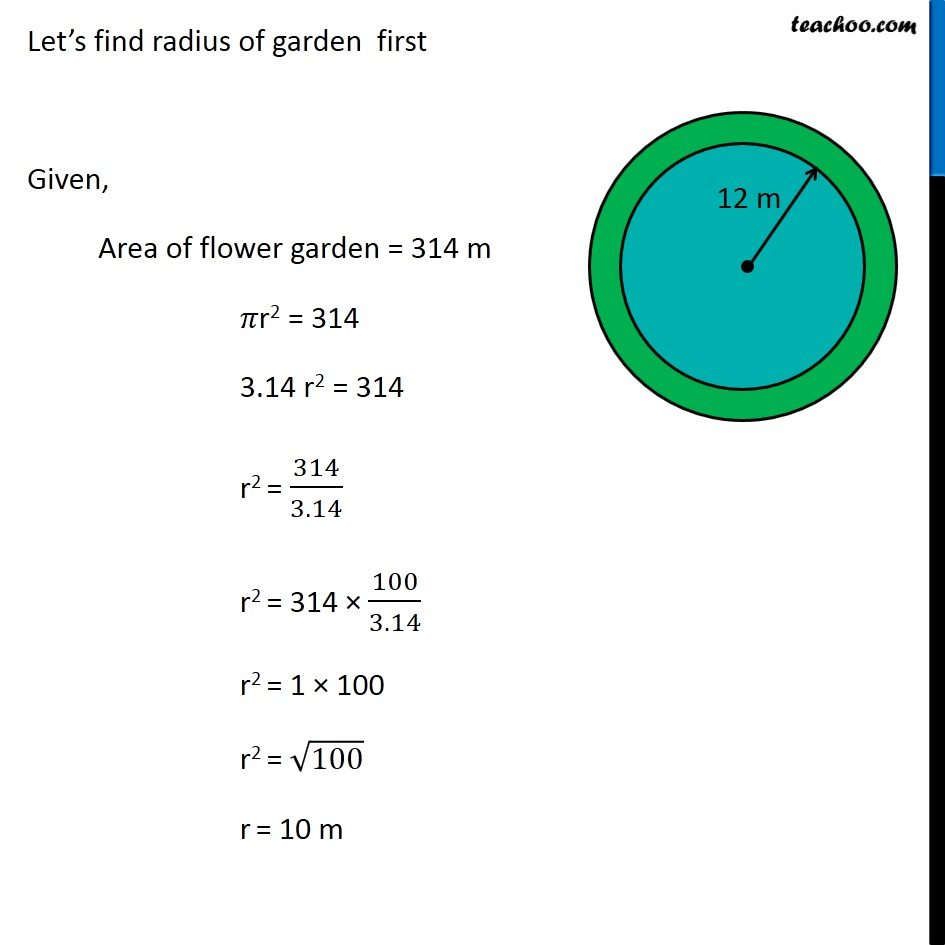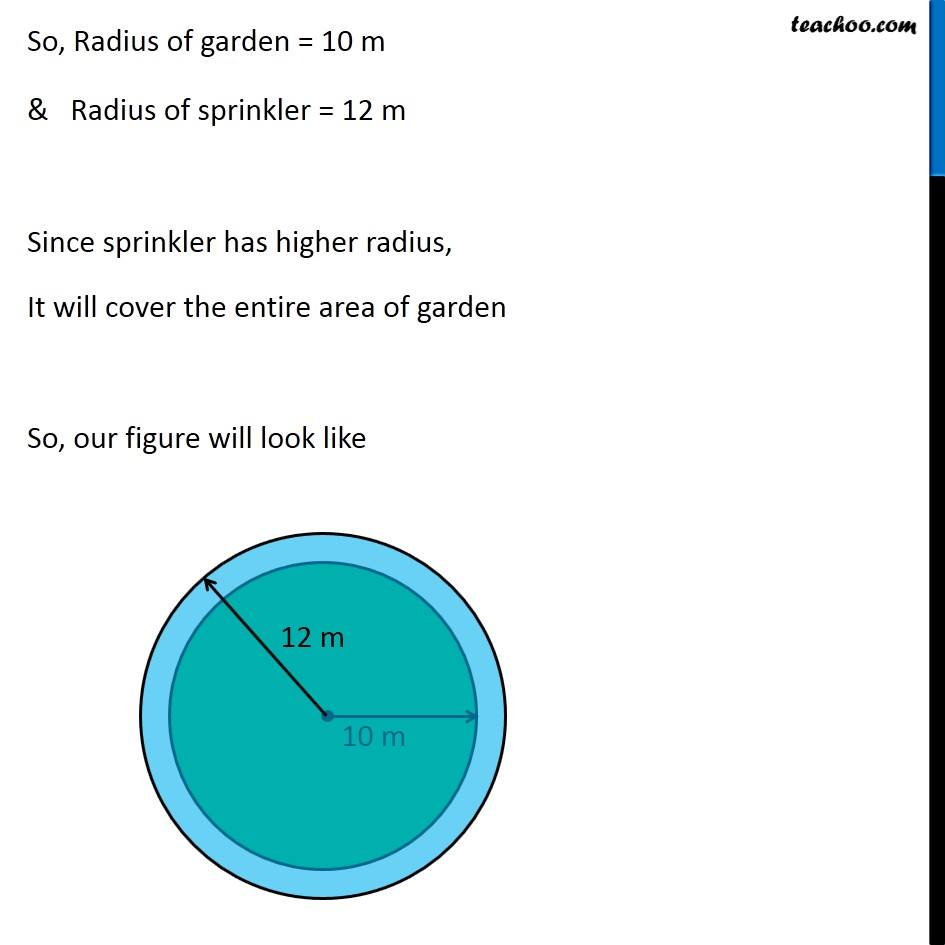Area of combination of figures - two circles, circle and square

Chapter 9 Class 7 Perimeter and Area
Concept wiseGet live Maths 1-on-1 Classs - Class 6 to 12

### Transcript

Ex 9.2, 14 A circular flower garden has an area of 314 m2. A sprinkler at the centre of the garden can cover an area that has a radius of 12 m. Will the sprinkler water the entire garden? (Take π = 3.14) We have a garden of area 314 m2 There is a sprinkler in the center, which covers area of circle with radius 12 m We need to find whether sprinkler can cover the entire garden Let’s find radius of garden first Given, Area of flower garden = 314 m 𝜋r2 = 314 3.14 r2 = 314 r2 = 314/3.14 r2 = 314 × 100/3.14 r2 = 1 × 100 r2 = √100 r = 10 m So, Radius of garden = 10 m & Radius of sprinkler = 12 m Since sprinkler has higher radius, It will cover the entire area of garden So, our figure will look like